Maxime Sangnier

September, 2020

# Sampling and testing ¶

## Random sampling ¶

### Simple random data¶

Numpy offers several routines to generate easily uniform and normal random samples:

In :
import numpy as np
import numpy.random as rdm
np.set_printoptions(precision=2)

rdm.rand(4, 4)  # Uniform sampling

Out:
array([[0.65, 0.82, 0.23, 0.23],
[0.44, 0.44, 0.32, 0.41],
[0.43, 0.96, 0.48, 0.85],
[0.93, 0.28, 0.98, 0.05]])
In :
rdm.randn(4, 4)  # Standard normal sampling

Out:
array([[ 0.53,  0.6 ,  0.98,  0.55],
[-1.14,  1.24,  0.81,  0.43],
[-0.16, -1.42,  1.14, -0.17],
[ 1.4 , -0.08,  1.94,  1.55]])
In :
rdm.randint(0, 10, size=(2, 3))  # Discrete uniform sampling (10 exclusive)

Out:
array([[3, 6, 7],
[9, 1, 1]])

In the case where the sampled integers are supposed to index an array, one can replace:

In :
a = np.arange(10)*10

ind = rdm.randint(0, 5, size=7)
a[ind]

Out:
array([20,  0, 10,  0, 40,  0,  0])

by:

In :
rdm.choice(a, size=7)

Out:
array([10, 80,  0, 70, 10,  0,  0])

The previous routines sample integers with replacement. To sample without replacement, one can use:

In :
rdm.permutation(10)[:7]  # 7 first items of a random permutation of [0, …, 9]

Out:
array([1, 8, 3, 0, 2, 9, 7])

Note that one can also directly permute an array with a copy (permutation) or in-place (shuffle), instead of generating random indexes.

In :
rdm.permutation(a)

Out:
array([40, 10, 20, 50, 80, 70, 30,  0, 60, 90])
In :
rdm.shuffle(a)
a

Out:
array([30, 50, 10, 60, 80, 40, 90, 70,  0, 20])

Question

Draw a sample of size $100$ from a normal distribution with mean $10$ and standard deviation $2$. Print the usual estimators of these two parameters to validate your operation.

In [ ]:
# Answer


### Random generator¶

It is obvious that random generators used in scientific computing are in fact pseudo-random generators. As a consequence, the practitioner is able to control them to a certain extent. In a way, this is good news for reproducible science!

Both examples below illustrate how to replay a random sampling.

In :
for it in range(3):
rdm.seed(it)  # Seed the generator to the current iteration number
print(rdm.randint(100, size=3))

[44 47 64]
[37 12 72]
[40 15 72]

In :
for it in range(3):
rdm.seed(it)  # Seed the generator to the current iteration number
print(rdm.randint(100, size=3))  # Same as before!

[44 47 64]
[37 12 72]
[40 15 72]

In :
s = rdm.get_state()  # Get the internal state of the generator
print(np.array([rdm.randn() for it in range(3)]))
print(rdm.rand(3, 3))

[-2.14  1.64 -1.79]
[[0.2  0.62 0.3 ]
[0.27 0.62 0.53]
[0.13 0.51 0.18]]

In :
rdm.set_state(s)  # Set the internal state of the generator to its previous value
for it in range(3):
print(rdm.randn(1))  # Same as before!

[-2.14]
[1.64]
[-1.79]


### Distributions¶

Besides the previous routines, Numpy offers the possibility to draw samples from numerous distributions.

In :
x = rdm.poisson(lam=4, size=500)
print(x[:30])

[3 4 5 2 2 2 4 6 6 3 2 1 6 2 5 3 3 5 3 6 7 6 5 3 2 3 2 4 4 3]

In :
import matplotlib.pyplot as plt
import seaborn as sns
sns.set()

fig, ax = plt.subplots()
ax.stem(np.bincount(x), use_line_collection=True);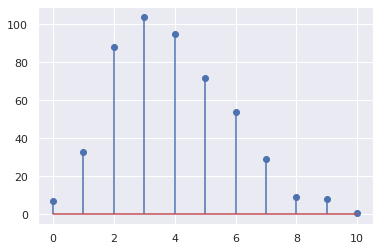Question

Draw a sample of size $1000$ from an exponential distribution with scale $2$ and plot a density histogram of this sample.

In [ ]:
# Answer


### Special functions¶

Many raw statistical routines (cumulative, survival and inverse functions) are available in the scipy.special mudule.

In :
from scipy import special

plt.stem(special.pdtr(range(15), 4), use_line_collection=True);  # Poisson cumulative distribution function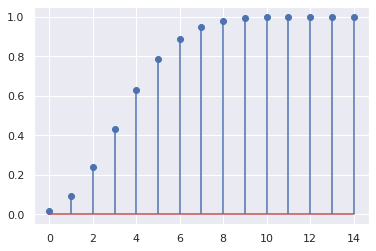### Random variables¶

Scipy.stats implements random variables with two different classes: continuous random variables and discrete random variables. As an example, we focus here on the gamma distribution and illustrate the main methods available.

In :
from scipy.stats import gamma

# gamma is a r.v. object corresponding to the standard gamma distribution
print("Distribution support: [{}, {}]".format(gamma.a, gamma.b))
print("Number of shape parameters: {} (name: {})".format(gamma.numargs, gamma.shapes))

Distribution support: [0.0, inf]
Number of shape parameters: 1 (name: a)


The shape parameter a appears in the probability density function: $$f(x; a) = \frac{x^{a-1}\exp(-x)}{\Gamma(a)}.$$

Since the shape parameter a is required, one has to specify it for each method.

In :
print("Mean:", gamma.mean(a=4))
print("Median:", gamma.median(a=4))
print("Variance:", gamma.var(a=4))

Mean: 4.0
Median: 3.672060748850897
Variance: 4.0


Two other parameters can be passed to the methods: loc and scale. They correspond to shifting and rescaling the standard random variable with $x \mapsto scale \cdot x + loc.$

In :
print("Mean:", gamma.mean(a=4, loc=2, scale=0.1))

Mean: 2.4


Since passing those parameters time and again can become quite bothersome, one can freeze the parameters:

In :
rv = gamma(a=4, loc=2, scale=2)
print("Mean:", rv.mean())

Mean: 10.0


Now, let us have a look at the available methods.

In :
print(rv.rvs(size=10))  # Draw a random sample

[ 6.56 17.46 24.21  7.11  9.63  5.71  8.27  7.46  9.37 14.17]

In :
for n in range(4):
print("Moment {}:".format(n), rv.moment(n))

Moment 0: 1.0
Moment 1: 10.0
Moment 2: 116.0
Moment 3: 1544.0

In :
x = np.linspace(0, 30, num=50)

fig, ax = plt.subplots()
ax.plot(x, rv.pdf(x), label='pdf')  # Probability density function
ax.plot(x, rv.cdf(x)/10, label='cdf / 10')  # Cumulative density function
ax.legend(loc="best");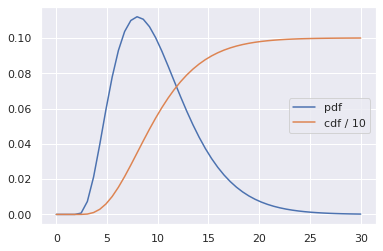In :
fig, ax = plt.subplots()
ax.plot(x, rv.logpdf(x), label='log pdf');  # Log of the pdf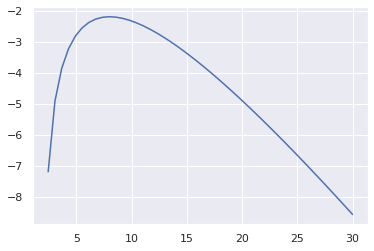In :
x = np.linspace(0, 1, num=50)

fig, ax = plt.subplots()
ax.plot(x, rv.ppf(x), label='ppf')  # Percent point function (inverse of cdf, also called quantile function)
ax.legend(loc="upper left");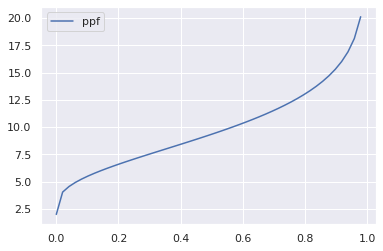In :
m = rv.expect(lambda x: np.exp(-x))  # Expectation of a function
print("First exponential moment: {0:0.2e}".format(m))

First exponential moment: 1.67e-03


Note that an unfrozen random variable (for instance gamma, not rv) benefits from two other methods: fit and fit_loc_scale for estimating parameters respectively by likelihood maximization and the moment method.

Question

Draw a sample of size $1000$ from a Laplace distribution (with location $1$ and scale $2$) and plot a density histogram of this sample. Add the curve of the probability density function.

In [ ]:
# Answer


## Descriptive statistics ¶

### Order statistics, moments and correlation¶

Basic descriptive statistics such as min, max, mean, median, std, variance and percentiles can be computed with array methods or routines from Numpy. Other empirical statistics such as mode and moments can be obtained with Scipy statistical functions.

### Histograms¶

A common task in statisics is to estimate the probability density function of a random variable, what is called density estimation. In a first approach, this task can be achieved by computing a histogram.

In :
x = rv.rvs(size=1000)  # Draw a random sample

# Compute the histogram
hist, bins = np.histogram(x, bins=20, density=True)

# Plot the histogram
fig, ax = plt.subplots()
ax.bar(bins[:-1], hist, width=bins-bins)
ax.set_xticks(bins[::3], ["{0:0.2f}".format(t) for t in bins[::3]]);

x_pdf = np.linspace(0, 30, num=100)
ax.plot(x_pdf, rv.pdf(x_pdf), color="red", linewidth=2, label="pdf")
ax.legend();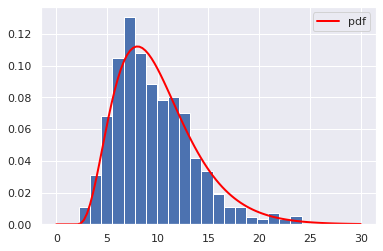Alternatively, one can use Matplotlib to produce fancy plots:

In :
fig, ax = plt.subplots()
hist_plt, bins_plt, patches = ax.hist(x, bins=20, density=True)
ax.plot(x_pdf, rv.pdf(x_pdf), color="red", linewidth=2, label="pdf")
ax.legend();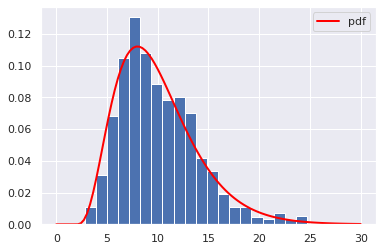### Kernel density estimation¶

Kernel desity estimation is a tool more efficient than a histogram for density estimation. In Python, kernel density estimation can be performed with the gaussian_kde function.

In :
from scipy.stats import gaussian_kde

kde = gaussian_kde(x)

fig, ax = plt.subplots()
(hist_plt, bins_plt, patches) = ax.hist(x, bins=20, density=True)
ax.plot(x_pdf, rv.pdf(x_pdf), color="red", linewidth=2, label="pdf")
ax.plot(x_pdf, kde(x_pdf), color="magenta", linewidth=2, label="kde")
ax.legend();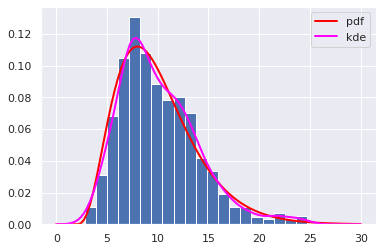Question Add on the previous figure the curves of a kernel density estimation with parameter bw_method (of gaussian_kde) varying according to bw_values.

In :
bw_values = [0.1, 0.5, 1]

In [ ]:
# Answer


## Hypothesis testing ¶

### Analyzing one sample¶

As for R, many tests can be performed in Python. For instance, a single sample may be analyzed with:

• ttest_1samp: T-test for the mean of one group of scores;
• kstest: Kolmogorov-Smirnov test for goodness of fit;
• ksone: general Kolmogorov-Smirnov one-sided test;
• chisquare: one-way chi square test;
• anderson: Anderson-Darling test for data coming from a particular distribution.
In :
from scipy import stats

for rv in [stats.expon, stats.norm]:
tt, pval = stats.ttest_1samp(rv.rvs(size=100), 0)
print("{} sample: ".format(rv.name), end="")
print("p-value = {0:0.2f} (statistics = {1:0.2f})".format(pval, tt))

expon sample: p-value = 0.00 (statistics = 10.52)
norm sample: p-value = 0.08 (statistics = -1.77)


On the one hand, the p-value for the exponential sample is small enough that we can reject the null hypothesis that the mean is $0$. On the other hand, with high significance levels, we cannot reject the null hypothesis for the normal sample (this is comforting!).

Let us now test for a given distribution:

In :
for rv in [stats.expon, stats.norm]:
tt, pval = stats.kstest(rv.rvs(size=100), 'expon')
print("{} sample: ".format(rv.name), end="")
print("p-value = {0:0.2f} (statistics = {1:0.2f})".format(pval, tt))

expon sample: p-value = 0.14 (statistics = 0.11)
norm sample: p-value = 0.00 (statistics = 0.56)


Again, the result is as expected.

### Testing normality¶

As a special case, several tests exist for assessing the normality of a sample:

• kurtosistest: tests whether a dataset has normal kurtosis;
• skewtest: tests whether the skew is different from the normal distribution;
• normaltest: tests whether a sample differs from a normal distribution (D'Agostino and Pearson's test);
• jarque_bera: Jarque-Bera goodness of fit test on sample data;
• shapiro: Shapiro-Wilk test for normality.
In :
for rv in [stats.expon, stats.norm]:
print("{} sample:".format(rv.name))
for name, test in [('skew', stats.skewtest), ('kurtosis', stats.kurtosistest)]:
tt, pval = test(rv.rvs(size=100))
print("   {} test: ".format(name), end="")
print("   p-value = {0:0.2f} (statistics = {1:0.2f})".format(pval, tt))

expon sample:
skew test:    p-value = 0.00 (statistics = 5.06)
kurtosis test:    p-value = 0.05 (statistics = 1.99)
norm sample:
skew test:    p-value = 0.46 (statistics = -0.74)
kurtosis test:    p-value = 0.93 (statistics = 0.09)


Note that these two tests are combined in the normality test:

In :
for rv in [stats.expon, stats.norm]:
tt, pval = stats.normaltest(rv.rvs(size=100))
print("{} sample: ".format(rv.name), end="")
print("p-value = {0:0.2f} (statistics = {1:0.2f})".format(pval, tt))

expon sample: p-value = 0.00 (statistics = 37.58)
norm sample: p-value = 0.51 (statistics = 1.36)


Question

Dowload this data file. Load the random sample stored in the variable x and test its normality.

In :
from scipy.io import loadmat


In [ ]:
# Answer


### Comparing two samples¶

Again, many tests for two samples are available in the scipy.stats module:

• ks_2samp: Kolmogorov-Smirnov test for 2 samples;
• ttest_ind: T-test for the means of two independent samples of scores;
• kstwobign: Kolmogorov-Smirnov two-sided test for large N;
• ttest_ind_from_stats: T-test for means of two independent samples from descriptive statistics;
• ttest_rel: T-test on TWO RELATED samples of scores, a and b;
• mannwhitneyu: Mann-Whitney rank test on samples x and y;
• wilcoxon: Wilcoxon signed-rank test;
• kruskal: Kruskal-Wallis H-test for independent samples;
• ansari: Ansari-Bradley test for equal scale parameters;
• bartlett: Bartlett's test for equal variances;
• levene: Levene test for equal variances;
• anderson_ksamp: Anderson-Darling test for k-samples;
• fligner: Fligner-Killeen test for equality of variances;
• median_test: Mood's median test;
• mood: Mood's test for equal scale parameters.

As an example, on can test that two independent samples have identical means or that two independent samples are drawn from the same continuous distribution:

In :
rvs1 = stats.norm.rvs(size=100, loc=0., scale=1)
rvs2 = stats.norm.rvs(size=200, loc=0.1, scale=2)

tt, pval = stats.ttest_ind(rvs1,rvs2)
print("p-value = {0:0.2f} (statistics = {1:0.2f})".format(pval, tt))

p-value = 0.34 (statistics = -0.95)


Here, the p-value is high enough that we cannot reject the null hypothesis that the two samples have identical means. On the other hand, the following statistical test makes it possible to state that the two samples are drawn from different distribution (we reject the null hypothesis that they come from the same distribution):

In :
tt, pval = stats.ks_2samp(rvs1, rvs2)
print("p-value = {0:0.2f} (statistics = {1:0.2f})".format(pval, tt))

p-value = 0.00 (statistics = 0.26)


Question

Apply both T-tests ttest_ind and ttest_rel on the samples rvs1 and rvs1_coupled. Can you explain the difference in the results?

In :
rvs1_coupled = rvs1 + rdm.randn(rvs1.size) * 0.1 + 0.1
print(rvs1.mean(), rvs1_coupled.mean())

-0.054561153736931295 0.05255814305890341

In [ ]:
# Answer


### Other tests¶

• pearsonr: Pearson correlation coefficient and the p-value for testing non-correlation;
• spearmanr: Spearman rank-order correlation coefficient and the p-value to test for non-correlation;
• power_divergence: Cressie-Read power divergence statistic and goodness of fit test;
• friedmanchisquare: Friedman test for repeated measurements;
• chi2_contingency: Chi-square test of independence of variables in a contingency table;
• fisher_exact: Fisher exact test on a 2x2 contingency table.

# Data representation and manipulation ¶

Following the example of R, Python comes with a package for handling data as a table : the Pandas package provides a container for tables, called dataframe. A dataframe is a two-dimensional table, in which each column contains measurements on one variable, and each row contains one individual.

The main features of Pandas and its dataframe are:

• reading data from csv and Excel files;
• giving names to variables;
• storing in a clever manner a large amound of data;
• providing methods for descriptive statistics.

## Reading and creating a dataframe ¶

A dataframe may be either read from a file or created from raw data (the file is available here):

In :
!cat data/defra_consumption.csv

;England;Wales;Scotland;N Ireland
Cheese;105;103;103;66
Carcass meat;245;227;242;267
Other meat;685;803;750;586
Fish;147;160;122;93
Fats and oils;193;235;184;209
Sugars;156;175;147;139
Fresh potatoes;720;874;566;1033
Fresh Veg;253;265;171;143
Other Veg;488;570;418;355
Processed potatoes;198;203;220;187
Processed Veg;360;365;337;334
Fresh fruit;1102;1137;957;674
Cereals;1472;1582;1462;1494
Beverages;57;73;53;47
Soft drinks;1374;1256;1572;1506
Alcoholic drinks;375;475;458;135
Confectionery;54;64;62;41

In :
import numpy as np
import pandas as pd

consumption

Out:
England Wales Scotland N Ireland
Cheese 105 103 103 66
Carcass meat 245 227 242 267
Other meat 685 803 750 586
Fish 147 160 122 93
Fats and oils 193 235 184 209
Sugars 156 175 147 139
Fresh potatoes 720 874 566 1033
Fresh Veg 253 265 171 143
Other Veg 488 570 418 355
Processed potatoes 198 203 220 187
Processed Veg 360 365 337 334
Fresh fruit 1102 1137 957 674
Cereals 1472 1582 1462 1494
Beverages 57 73 53 47
Soft drinks 1374 1256 1572 1506
Alcoholic drinks 375 475 458 135
Confectionery 54 64 62 41
In :
timeseries = pd.DataFrame(data=np.random.randn(6,4),
index=pd.date_range('20130101', periods=6),
columns=list('ABCD'))
timeseries

Out:
A B C D
2013-01-01 -0.429630 2.257868 -0.765199 1.047689
2013-01-02 -0.897261 -1.181121 1.017547 1.058445
2013-01-03 1.075539 -0.549968 -1.587368 0.389060
2013-01-04 -1.595424 0.257599 0.202211 -2.483426
2013-01-05 -1.305641 0.480583 -0.135586 0.421599
2013-01-06 0.308346 -0.164241 0.446200 0.744765
In :
df = pd.DataFrame({'A' : 1.,  # Single item
'B' : pd.Timestamp('20130102'),  # Single item
'C' : np.random.randn(4),  # Multiple item
'D' : pd.Categorical(["test", "train", "test", "train"])})
df

Out:
A B C D
0 1.0 2013-01-02 -0.138763 test
1 1.0 2013-01-02 0.060227 train
2 1.0 2013-01-02 -0.429151 test
3 1.0 2013-01-02 1.244043 train

Question

Create a dataframe, the columns of which are entitled normal, exponential and laplace, and contain random samples of size $10$ of each distribution.

In [ ]:
# Answer


## Viewing data ¶

Since it is unrealistic to view a table in whole, Pandas provides different methods to give a sneak look at the aforesaid table.

In :
timeseries.index  # Index of the table

Out:
DatetimeIndex(['2013-01-01', '2013-01-02', '2013-01-03', '2013-01-04',
'2013-01-05', '2013-01-06'],
dtype='datetime64[ns]', freq='D')
In :
consumption.index  # Index of the table

Out:
Index(['Cheese', 'Carcass meat', 'Other meat', 'Fish', 'Fats and oils',
'Sugars', 'Fresh potatoes', 'Fresh Veg', 'Other Veg',
'Processed potatoes', 'Processed Veg', 'Fresh fruit', 'Cereals',
'Beverages', 'Soft drinks', 'Alcoholic drinks', 'Confectionery'],
dtype='object')
In :
consumption.columns  # Columns of the table

Out:
Index(['England', 'Wales', 'Scotland', 'N Ireland'], dtype='object')
In :
consumption.head(n=3)

Out:
England Wales Scotland N Ireland
Cheese 105 103 103 66
Carcass meat 245 227 242 267
Other meat 685 803 750 586
In :
consumption.tail(n=3)

Out:
England Wales Scotland N Ireland
Soft drinks 1374 1256 1572 1506
Alcoholic drinks 375 475 458 135
Confectionery 54 64 62 41
In :
consumption.values  # Values are in a Numpy array

Out:
array([[ 105,  103,  103,   66],
[ 245,  227,  242,  267],
[ 685,  803,  750,  586],
[ 147,  160,  122,   93],
[ 193,  235,  184,  209],
[ 156,  175,  147,  139],
[ 720,  874,  566, 1033],
[ 253,  265,  171,  143],
[ 488,  570,  418,  355],
[ 198,  203,  220,  187],
[ 360,  365,  337,  334],
[1102, 1137,  957,  674],
[1472, 1582, 1462, 1494],
[  57,   73,   53,   47],
[1374, 1256, 1572, 1506],
[ 375,  475,  458,  135],
[  54,   64,   62,   41]])

The methods info and describe give respectively general and quantitative information concerning the dataframe. In particular, info indicates the categorical variables (which are not treated by describe).

In :
df.info()

<class 'pandas.core.frame.DataFrame'>
RangeIndex: 4 entries, 0 to 3
Data columns (total 4 columns):
A    4 non-null float64
B    4 non-null datetime64[ns]
C    4 non-null float64
D    4 non-null category
dtypes: category(1), datetime64[ns](1), float64(2)
memory usage: 324.0 bytes

In :
df.describe()

Out:
A C
count 4.0 4.000000
mean 1.0 0.184089
std 0.0 0.734652
min 1.0 -0.429151
25% 1.0 -0.211360
50% 1.0 -0.039268
75% 1.0 0.356181
max 1.0 1.244043

If you suspect a variable to be categorical, you can state it.

In :
df['E'] = np.random.randint(0, high=2, size=df.shape)
df['E'] = df['E'].astype('category')
df

Out:
A B C D E
0 1.0 2013-01-02 -0.138763 test 0
1 1.0 2013-01-02 0.060227 train 1
2 1.0 2013-01-02 -0.429151 test 1
3 1.0 2013-01-02 1.244043 train 0
In :
df.info()

<class 'pandas.core.frame.DataFrame'>
RangeIndex: 4 entries, 0 to 3
Data columns (total 5 columns):
A    4 non-null float64
B    4 non-null datetime64[ns]
C    4 non-null float64
D    4 non-null category
E    4 non-null category
dtypes: category(2), datetime64[ns](1), float64(2)
memory usage: 424.0 bytes

In :
timeseries.sort_index(ascending=False)

Out:
A B C D
2013-01-06 0.308346 -0.164241 0.446200 0.744765
2013-01-05 -1.305641 0.480583 -0.135586 0.421599
2013-01-04 -1.595424 0.257599 0.202211 -2.483426
2013-01-03 1.075539 -0.549968 -1.587368 0.389060
2013-01-02 -0.897261 -1.181121 1.017547 1.058445
2013-01-01 -0.429630 2.257868 -0.765199 1.047689
In :
timeseries.sort_values(by='A')

Out:
A B C D
2013-01-04 -1.595424 0.257599 0.202211 -2.483426
2013-01-05 -1.305641 0.480583 -0.135586 0.421599
2013-01-02 -0.897261 -1.181121 1.017547 1.058445
2013-01-01 -0.429630 2.257868 -0.765199 1.047689
2013-01-06 0.308346 -0.164241 0.446200 0.744765
2013-01-03 1.075539 -0.549968 -1.587368 0.389060

Question

Print the first 10 lines of the dataframe consumption.

In [ ]:
# Answer


## Indexing a table ¶

### Natural indexing¶

Explanations are provided with the consumption dataframe.

In :
consumption.head()

Out:
England Wales Scotland N Ireland
Cheese 105 103 103 66
Carcass meat 245 227 242 267
Other meat 685 803 750 586
Fish 147 160 122 93
Fats and oils 193 235 184 209

Natural indexing is performed with []. This indexes the columns of dataframes and the rows of series.

In :
consumption['England']

Out:
Cheese                 105
Carcass meat           245
Other meat             685
Fish                   147
Fats and oils          193
Sugars                 156
Fresh potatoes         720
Fresh Veg              253
Other Veg              488
Processed potatoes     198
Processed Veg          360
Fresh fruit           1102
Cereals               1472
Beverages               57
Soft drinks           1374
Alcoholic drinks       375
Confectionery           54
Name: England, dtype: int64
In :
s = consumption['England']  # A series
s['Cheese']

Out:
105

You may want to extract several columns or several rows.

In :
consumption[['England', 'Scotland']].head()

Out:
England Scotland
Cheese 105 103
Carcass meat 245 242
Other meat 685 750
Fish 147 122
Fats and oils 193 184
In :
s[['Cheese', 'Other meat']]

Out:
Cheese        105
Other meat    685
Name: England, dtype: int64

Remark: selecting with [[]] always return a dataframe.

In :
consumption[['England']].head()

Out:
England
Cheese 105
Carcass meat 245
Other meat 685
Fish 147
Fats and oils 193

Question

Print together columns B and D of the dataframe df.

In [ ]:
# Answer


### Label based indexing¶

Label based indexing is an enhancement of natural indexing, accessible with .loc[]. Indexing has to be thought as a matrix but with labels instead of positions. Hence, the rows are indexed first (instead of the columns with []).

In :
consumption.loc['Cheese']  # Single row

Out:
England      105
Wales        103
Scotland     103
N Ireland     66
Name: Cheese, dtype: int64
In :
consumption.loc[:, 'England'].head()  # Single column

Out:
Cheese           105
Carcass meat     245
Other meat       685
Fish             147
Fats and oils    193
Name: England, dtype: int64
In :
consumption.loc[['Cheese', 'Fresh potatoes']]  # Multiple rows

Out:
England Wales Scotland N Ireland
Cheese 105 103 103 66
Fresh potatoes 720 874 566 1033

Slicing on rows and columns is possible but endpoints are included.

In :
consumption.loc['Cheese':'Fish']  # Row slicing

Out:
England Wales Scotland N Ireland
Cheese 105 103 103 66
Carcass meat 245 227 242 267
Other meat 685 803 750 586
Fish 147 160 122 93
In :
consumption.loc['Cheese':'Cereals':4]  # Row slicing (from Cheese to Cereals with step 2)

Out:
England Wales Scotland N Ireland
Cheese 105 103 103 66
Fats and oils 193 235 184 209
Other Veg 488 570 418 355
Cereals 1472 1582 1462 1494
In :
consumption.loc['Cheese':'Cereals':4, :'Wales']  # Row and column slicing

Out:
England Wales
Cheese 105 103
Fats and oils 193 235
Other Veg 488 570
Cereals 1472 1582

Question

What is the value in the dataframe timeseries at row 2013-01-04 and column B?

In [ ]:
# Answer


### Integer position based indexing¶

Interger location (or position) based indexing is done with .iloc[]. It is similar to .loc[] but consideres only integer positions instead of labels.

Remark: endpoints are not included (similarly to Numpy).

In :
consumption.iloc[:2]

Out:
England Wales Scotland N Ireland
Cheese 105 103 103 66
Carcass meat 245 227 242 267
In :
consumption.iloc[10::4, ::2]

Out:
England Scotland
Processed Veg 360 337
Soft drinks 1374 1572

### Boolean indexing¶

Similarly to Numpy arrays, dataframes can be indexed with Boolean variables thanks to .loc[].

In :
consumption.loc[consumption['England'] > 500]  # Row selection

Out:
England Wales Scotland N Ireland
Other meat 685 803 750 586
Fresh potatoes 720 874 566 1033
Fresh fruit 1102 1137 957 674
Cereals 1472 1582 1462 1494
Soft drinks 1374 1256 1572 1506
In :
consumption.loc[consumption['England'] > 500, consumption.loc['Other meat'] < 800]  # Row and column selection

Out:
England Scotland N Ireland
Other meat 685 750 586
Fresh potatoes 720 566 1033
Fresh fruit 1102 957 674
Cereals 1472 1462 1494
Soft drinks 1374 1572 1506

Operating on the whole dataframe puts NaN where the condition is not satisfied. Beyond that, it is useful for assignments.

In :
timeseries[timeseries > 0]  # Set NaN where condition is not satisfied

Out:
A B C D
2013-01-01 NaN 2.257868 NaN 1.047689
2013-01-02 NaN NaN 1.017547 1.058445
2013-01-03 1.075539 NaN NaN 0.389060
2013-01-04 NaN 0.257599 0.202211 NaN
2013-01-05 NaN 0.480583 NaN 0.421599
2013-01-06 0.308346 NaN 0.446200 0.744765
In :
tt = timeseries.copy()
tt[tt < 0] = 0
tt

Out:
A B C D
2013-01-01 0.000000 2.257868 0.000000 1.047689
2013-01-02 0.000000 0.000000 1.017547 1.058445
2013-01-03 1.075539 0.000000 0.000000 0.389060
2013-01-04 0.000000 0.257599 0.202211 0.000000
2013-01-05 0.000000 0.480583 0.000000 0.421599
2013-01-06 0.308346 0.000000 0.446200 0.744765

The isin method enables selecting with an OR condition.

In :
df.loc[df['D'].isin(['test', 'train'])]

Out:
A B C D E
0 1.0 2013-01-02 -0.138763 test 0
1 1.0 2013-01-02 0.060227 train 1
2 1.0 2013-01-02 -0.429151 test 1
3 1.0 2013-01-02 1.244043 train 0

Question

Select rows of df with $1$ in column E.

In [ ]:
# Answer


### Strange behaviors of natural indexing I do not recommend¶

Here are some strange and confusing behaviors of Pandas objects.

I advise you not to use those features, but to adopt .loc[] and .iloc[] instead.

Series (not dataframes) can be indexed with integer numbers.

In :
s

Out:
105

And sliced… with endpoints excluded.

In :
s[0:2]  # 0: Cheese, 1: Carcass meat, 2: Other meat

Out:
Cheese          105
Carcass meat    245
Name: England, dtype: int64

Slicing is also possible with labels… with endpoints included.

In :
s['Cheese':'Other meat']

Out:
Cheese          105
Carcass meat    245
Other meat      685
Name: England, dtype: int64

You can also slice rows of a dataframe using the same notation (while one would expect to slice columns). Even stranger, slicing works with integer positions (for example consumption[2:5]), still with endpoints included with labels and excluded with integer positions, but not single indexing (consumption raises an error).

In :
consumption['Cheese':'Cereals':2]

Out:
England Wales Scotland N Ireland
Cheese 105 103 103 66
Other meat 685 803 750 586
Fats and oils 193 235 184 209
Fresh potatoes 720 874 566 1033
Other Veg 488 570 418 355
Processed Veg 360 365 337 334
Cereals 1472 1582 1462 1494

Similarly to slicing, Boolean indexing operates on dataframe rows.

In :
consumption[consumption['England'] > 500]  # Similar to consumption.loc[consumption['England'] > 500]

Out:
England Wales Scotland N Ireland
Other meat 685 803 750 586
Fresh potatoes 720 874 566 1033
Fresh fruit 1102 1137 957 674
Cereals 1472 1582 1462 1494
Soft drinks 1374 1256 1572 1506

### Selection random samples¶

The sample method makes it possible to randomly select rows (individuals) from a dataframe (without replacement).

In :
consumption.sample(n=3)

Out:
England Wales Scotland N Ireland
Confectionery 54 64 62 41
Fresh Veg 253 265 171 143
Fresh fruit 1102 1137 957 674

## Adding and deleting items ¶

Let us consider a copy of the first 5 rows of consumption.

In :
cons = consumption.iloc[:5].copy()
cons

Out:
England Wales Scotland N Ireland
Cheese 105 103 103 66
Carcass meat 245 227 242 267
Other meat 685 803 750 586
Fish 147 160 122 93
Fats and oils 193 235 184 209

We successively add a column and a row to cons.

In :
cons['all'] = cons.sum(axis=1)
cons.loc['Chocolate'] = [70, 60, 60, 150, 340]
cons

Out:
England Wales Scotland N Ireland all
Cheese 105 103 103 66 377
Carcass meat 245 227 242 267 981
Other meat 685 803 750 586 2824
Fish 147 160 122 93 522
Fats and oils 193 235 184 209 821
Chocolate 70 60 60 150 340

That we can now drop.

In :
cons = cons.drop('Chocolate')
cons = cons.drop('all', axis=1)
cons

Out:
England Wales Scotland N Ireland
Cheese 105 103 103 66
Carcass meat 245 227 242 267
Other meat 685 803 750 586
Fish 147 160 122 93
Fats and oils 193 235 184 209

Dataframes can be concatenated along columns and rows.

In :
pd.concat((cons[['England']], cons[['Scotland']]), axis=1)

Out:
England Scotland
Cheese 105 103
Carcass meat 245 242
Other meat 685 750
Fish 147 122
Fats and oils 193 184
In :
pd.concat((cons.iloc[:2], cons.iloc[-2:]))

Out:
England Wales Scotland N Ireland
Cheese 105 103 103 66
Carcass meat 245 227 242 267
Fish 147 160 122 93
Fats and oils 193 235 184 209

This last operation is similar to:

In :
cons.iloc[:2].append(cons.iloc[-2:])

Out:
England Wales Scotland N Ireland
Cheese 105 103 103 66
Carcass meat 245 227 242 267
Fish 147 160 122 93
Fats and oils 193 235 184 209

## Managing missing data ¶

Missing data are generally replaced by a NaN in the table. Pandas offers several possibilities to manage them.

In :
cons_md = consumption[consumption>200]
cons_md.iloc[:, -1] = consumption.iloc[:, -1]
cons_md.head()  # A table with missing data

Out:
England Wales Scotland N Ireland
Cheese NaN NaN NaN 66
Carcass meat 245.0 227.0 242.0 267
Other meat 685.0 803.0 750.0 586
Fish NaN NaN NaN 93
Fats and oils NaN 235.0 NaN 209
In :
cons_md.dropna()  # Drop any row with missing data

Out:
England Wales Scotland N Ireland
Carcass meat 245.0 227.0 242.0 267
Other meat 685.0 803.0 750.0 586
Fresh potatoes 720.0 874.0 566.0 1033
Other Veg 488.0 570.0 418.0 355
Processed Veg 360.0 365.0 337.0 334
Fresh fruit 1102.0 1137.0 957.0 674
Cereals 1472.0 1582.0 1462.0 1494
Soft drinks 1374.0 1256.0 1572.0 1506
Alcoholic drinks 375.0 475.0 458.0 135
In :
cons_md.fillna(method='ffill').head()  # Fill missing data with previous real values
# This does not work with the first line

Out:
England Wales Scotland N Ireland
Cheese NaN NaN NaN 66
Carcass meat 245.0 227.0 242.0 267
Other meat 685.0 803.0 750.0 586
Fish 685.0 803.0 750.0 93
Fats and oils 685.0 235.0 750.0 209
In :
cons_md.fillna(method='ffill').fillna(method='bfill').head()

Out:
England Wales Scotland N Ireland
Cheese 245.0 227.0 242.0 66
Carcass meat 245.0 227.0 242.0 267
Other meat 685.0 803.0 750.0 586
Fish 685.0 803.0 750.0 93
Fats and oils 685.0 235.0 750.0 209
In :
cons_md.isnull().any()  # Test for missing data

Out:
England       True
Wales         True
Scotland      True
N Ireland    False
dtype: bool

Question

By changing the axis parameter of dropna, drop the columns with missing values of cons_md.

In [ ]:
# Answer


## Descriptive statistics ¶

A dataframe comes with many methods for descriptive statistics:

• count: Number of non-null observations;
• sum: Sum of values;
• mean: Mean of values;
• mad: Mean absolute deviation;
• median: Arithmetic median of values;
• min: Minimum;
• max: Maximum;
• mode: Mode;
• abs: Absolute Value;
• prod: Product of values;
• std: Bessel-corrected sample standard deviation;
• var: Unbiased variance;
• sem: Standard error of the mean;
• skew: Sample skewness (3rd moment);
• kurt: Sample kurtosis (4th moment);
• quantile: Sample quantile (value at %);
• cumsum: Cumulative sum;
• cumprod: Cumulative product;
• cummax: Cumulative maximum;
• cummin: Cumulative minimum.
In :
df.median()  # Median of numeric columns

Out:
A    1.000000
C   -0.039268
E    0.500000
dtype: float64
In :
df.median(axis=1)  # Median of rows (numeric objects only)

Out:
0    0.430619
1    0.530114
2    0.285425
3    1.122021
dtype: float64
In :
df['D'].value_counts()  # Histrogramming

Out:
train    2
test     2
Name: D, dtype: int64

Question

Compute the cumulative sum of the consumption data.

In [ ]:
# Answer


## Pivot table ¶

A pivot table aggregates the values of a column according to the similar values of other columns (which will become the row indexes and columns labels of the aggregated table).

In :
df['E'] = ['success', 'failure', 'failure', 'success']
df

Out:
A B C D E
0 1.0 2013-01-02 -0.138763 test success
1 1.0 2013-01-02 0.060227 train failure
2 1.0 2013-01-02 -0.429151 test failure
3 1.0 2013-01-02 1.244043 train success
In :
# Pivot table:
# values: label of the column whose values will be reorganized and displayed in the table
# index: indexes of the final table
# columns: columns of the final table
pd.pivot_table(df, values='C', index='D', columns='E')

Out:
E failure success
D
test -0.429151 -0.138763
train 0.060227 1.244043

## Plotting ¶

### With pandas¶

Pandas provides a rich collection of techniques to vizualize dataframes. Here, we illustrate just a few of them.

In :
consumption.plot(figsize=(10, 6));  # Columns vs index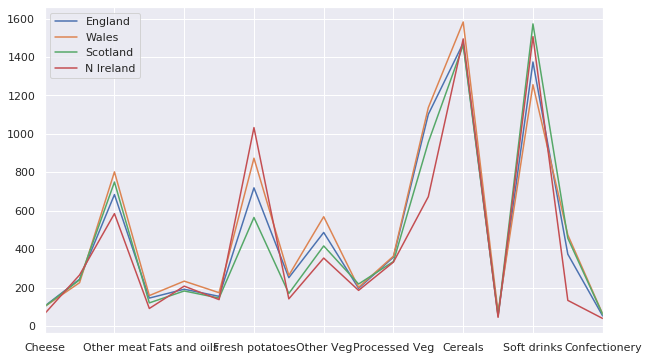In :
consumption.plot(subplots=True, figsize=(10, 8));  # Columns vs index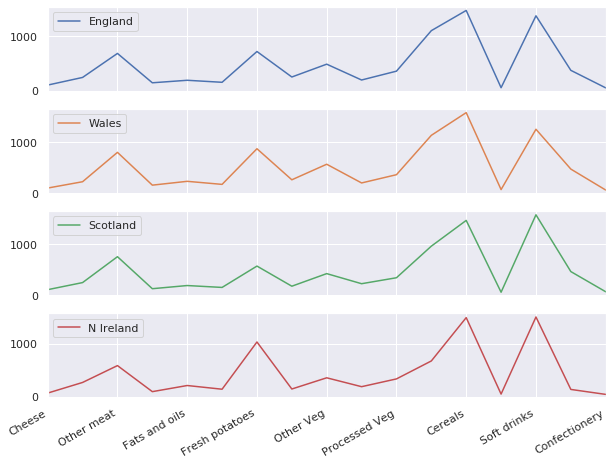In :
consumption.plot.barh(stacked=True, figsize=(10, 6));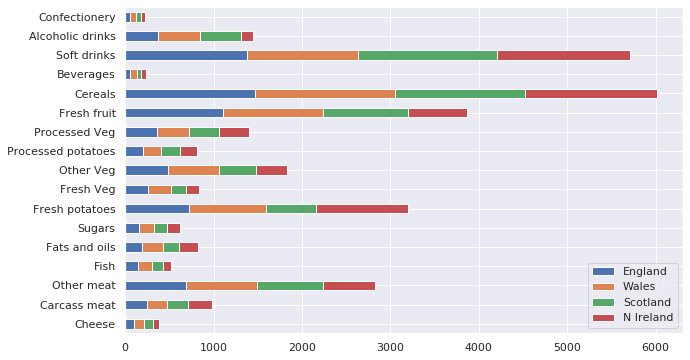Question

Plot a pie chart (parameter kind='pie' of plot) of the average consumption of foodstuff.

In [ ]:
# Answer


### With Seaborn¶

Besides its esthetics purpose, Seaborn provides many routines for producing beautiful plots from dataframes, particularly for statistical data.

In :
fig, ax = plt.subplots(figsize=(10, 6))
sns.lineplot(x='England', y='Scotland', data=consumption);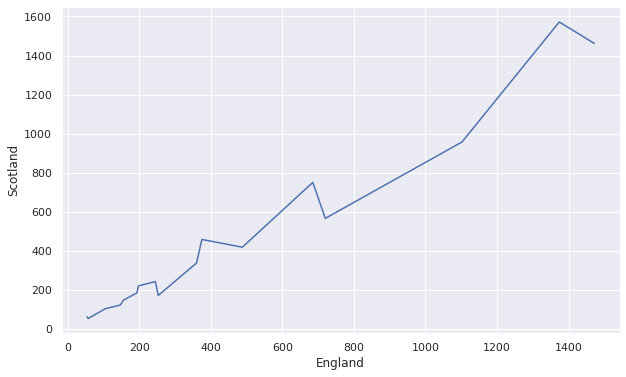In :
tips = sns.load_dataset('tips')

Out:
total_bill tip sex smoker day time size
0 16.99 1.01 Female No Sun Dinner 2
1 10.34 1.66 Male No Sun Dinner 3
2 21.01 3.50 Male No Sun Dinner 3
3 23.68 3.31 Male No Sun Dinner 2
4 24.59 3.61 Female No Sun Dinner 4
In :
import warnings
warnings.filterwarnings("ignore")

fig, ax = plt.subplots(figsize=(10, 6))
sns.violinplot(x='day', y='total_bill', hue='smoker', split=True, data=tips);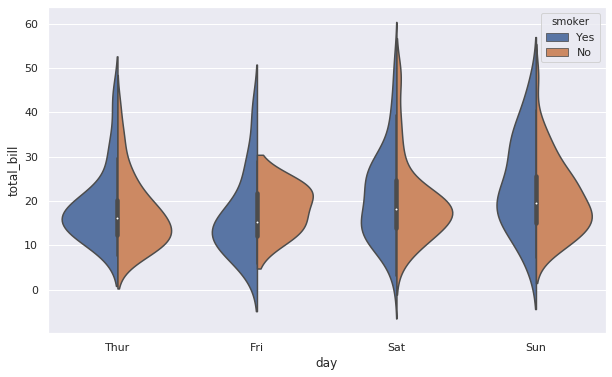In :
fig, ax = plt.subplots(figsize=(10, 6))
sns.barplot(x='day', y='tip', hue='time', data=tips)

Out:
<matplotlib.axes._subplots.AxesSubplot at 0x7fb45d0f8dd8>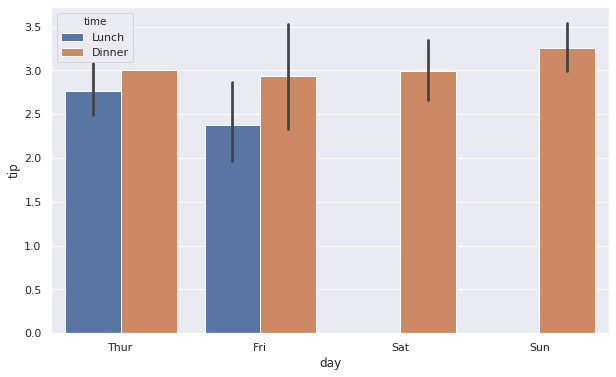# Linear models ¶

StatsModels is a scientific module based on Pandas for performing statistical analyses in Python. It provides tools for conducting data exploration, statistical tests and for the estimation of several statistical models. As a statistical package, each estimator in StatsModels comes with an extensive list of resulting statistics.

## Linear regression ¶

We illustrate here a major feature of StatsModels, which is linear regression.

### Simple example¶

In :
import statsmodels.api as sm
from statsmodels.sandbox.regression.predstd import wls_prediction_std

# Generate data
nsample = 50
sig = 0.5
x = np.linspace(0, 20, nsample)
X = np.column_stack((x, np.sin(x), (x-5)**2, np.ones(nsample)))
beta = [0.5, 0.5, -0.02, 5.]

y_true = np.dot(X, beta)
y = y_true + sig * np.random.normal(size=nsample)

y[np.random.permutation(y.size)[:3]] += 4 * (-1)**np.random.randint(0, 2, size=3)  # 3 outliers

In :
# Fit the model
model = sm.OLS(y, X)
res = model.fit()
res.summary()

Out:
Dep. Variable: R-squared: y 0.751 OLS 0.734 Least Squares 46.15 Tue, 22 Sep 2020 6.45e-14 16:59:26 -73.064 50 154.1 46 161.8 3 nonrobust
coef std err t P>|t| [0.025 0.975] 0.4958 0.057 8.672 0.000 0.381 0.611 0.7785 0.225 3.464 0.001 0.326 1.231 -0.0205 0.005 -4.093 0.000 -0.031 -0.010 5.1553 0.371 13.906 0.000 4.409 5.902
 Omnibus: Durbin-Watson: 41.161 2.011 0 345.917 1.658 7.67e-76 15.452 221

Warnings:
 Standard Errors assume that the covariance matrix of the errors is correctly specified.

Main attributes of the fitted model are:

In :
print('Parameters: ', res.params)
print('Standard errors: ', res.bse)
print('Predicted values: ', res.fittedvalues)

Parameters:  [ 0.5   0.78 -0.02  5.16]
Standard errors:  [0.06 0.22 0.01 0.37]
Predicted values:  [ 4.64  5.23  5.77  6.2   6.51  6.68  6.73  6.7   6.62  6.55  6.53  6.62
6.82  7.14  7.56  8.04  8.54  8.99  9.37  9.62  9.74  9.73  9.62  9.44
9.26  9.11  9.05  9.1   9.27  9.55  9.9  10.29 10.67 10.97 11.17 11.24
11.17 10.99 10.73 10.44 10.16  9.96  9.85  9.87 10.   10.23 10.51 10.79
11.02 11.16]


A method called predict is also available for prediction with the estimator:

In :
res.predict(X)  # Same as res.fittedvalues

Out:
array([ 4.64,  5.23,  5.77,  6.2 ,  6.51,  6.68,  6.73,  6.7 ,  6.62,
6.55,  6.53,  6.62,  6.82,  7.14,  7.56,  8.04,  8.54,  8.99,
9.37,  9.62,  9.74,  9.73,  9.62,  9.44,  9.26,  9.11,  9.05,
9.1 ,  9.27,  9.55,  9.9 , 10.29, 10.67, 10.97, 11.17, 11.24,
11.17, 10.99, 10.73, 10.44, 10.16,  9.96,  9.85,  9.87, 10.  ,
10.23, 10.51, 10.79, 11.02, 11.16])
In :
# Plot the regression
prstd, iv_l, iv_u = wls_prediction_std(res)  # Curves for standard deviation

fig, ax = plt.subplots()
ax.plot(x, y, 'o', label="data")
ax.plot(x, y_true, 'b-', label="True")
ax.plot(x, res.fittedvalues, 'r--.', label="OLS")
ax.plot(x, iv_u, 'r--')
ax.plot(x, iv_l, 'r--')
ax.legend(loc='best');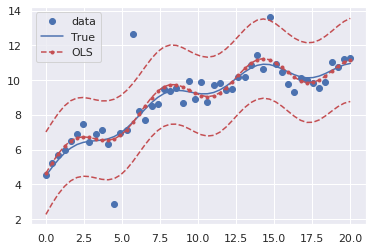In :
residuals = y - res.predict(X)  # Same as res.resid
print(residuals)

[-9.56e-02  3.60e-04 -5.25e-02 -2.06e-01 -2.61e-02  2.10e-01  7.19e-01
-2.71e-01  2.62e-01  5.74e-01 -1.89e-01 -3.74e+00  1.43e-01 -3.85e-02
5.10e+00  1.58e-01 -8.59e-01 -5.07e-01 -7.50e-01 -1.20e-01 -3.58e-01
-1.74e-01 -9.44e-01  4.75e-01 -3.43e-01  7.70e-01 -2.83e-01  6.08e-01
5.87e-01 -1.29e-01 -4.30e-01 -1.33e-01 -4.77e-01 -1.08e-01  2.57e-01
-6.15e-01  2.47e+00 -7.23e-02 -2.46e-01 -6.36e-01 -8.28e-01  1.71e-01
1.68e-01 -3.14e-02 -4.50e-01 -3.48e-01  5.32e-01 -2.21e-02  1.70e-01
9.64e-02]


The sum of squared residuals (or residual sum of squares) is:

In :
print(np.sum(res.resid**2), res.ssr)

54.4182341449693 54.41823414496929


while an unbiased estimate of the variance is:

In :
print(res.ssr / res.df_resid, res.scale)

1.183005090108028 1.183005090108028


The hat (or projection matrix) is:

In :
H = X.dot(np.linalg.solve(X.T.dot(X), X.T))


Then, the studentized residuals are:

In :
t = res.resid / np.sqrt(res.scale*(1-np.diag(H)))  # Standardized residuals
ts = t * np.sqrt( (res.df_resid-1) / (res.df_resid-t**2))  # Studentized residuals
print(ts)

[-9.57e-02  3.53e-04 -5.11e-02 -2.00e-01 -2.51e-02  2.00e-01  6.81e-01
-2.53e-01  2.45e-01  5.41e-01 -1.79e-01 -4.20e+00  1.36e-01 -3.63e-02
6.77e+00  1.47e-01 -8.03e-01 -4.76e-01 -7.12e-01 -1.14e-01 -3.40e-01
-1.64e-01 -8.91e-01  4.43e-01 -3.20e-01  7.26e-01 -2.68e-01  5.78e-01
5.56e-01 -1.20e-01 -4.00e-01 -1.23e-01 -4.46e-01 -1.02e-01  2.45e-01
-5.87e-01  2.49e+00 -6.76e-02 -2.28e-01 -5.93e-01 -7.80e-01  1.62e-01
1.60e-01 -3.02e-02 -4.33e-01 -3.33e-01  5.12e-01 -2.14e-02  1.68e-01
9.77e-02]


The studentized residuals can be directly obtained by:

In :
print(res.outlier_test()[:, 0])

[-9.57e-02  3.53e-04 -5.11e-02 -2.00e-01 -2.51e-02  2.00e-01  6.81e-01
-2.53e-01  2.45e-01  5.41e-01 -1.79e-01 -4.20e+00  1.36e-01 -3.63e-02
6.77e+00  1.47e-01 -8.03e-01 -4.76e-01 -7.12e-01 -1.14e-01 -3.40e-01
-1.64e-01 -8.91e-01  4.43e-01 -3.20e-01  7.26e-01 -2.68e-01  5.78e-01
5.56e-01 -1.20e-01 -4.00e-01 -1.23e-01 -4.46e-01 -1.02e-01  2.45e-01
-5.87e-01  2.49e+00 -6.76e-02 -2.28e-01 -5.93e-01 -7.80e-01  1.62e-01
1.60e-01 -3.02e-02 -4.33e-01 -3.33e-01  5.12e-01 -2.14e-02  1.68e-01
9.77e-02]

In :
fig, ax = plt.subplots()
ax.plot(ts, '.')
ax.plot([0, X.shape], [-2]*2, 'r')
ax.plot([0, X.shape], *2, 'r')

outliers = np.where(res.outlier_test()[:, -1]<0.1)
ax.plot(outliers, res.outlier_test()[outliers, 0], 'ro')

Out:
[<matplotlib.lines.Line2D at 0x7fb45d028710>,
<matplotlib.lines.Line2D at 0x7fb45cfbfcf8>]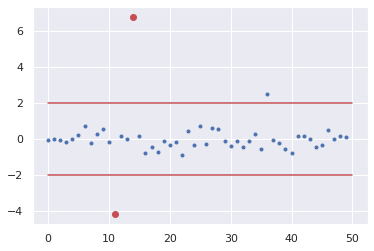Note that studentized residuals are only asymptotically normal.

In :
from scipy.stats import normaltest, probplot

print(normaltest(ts))

NormaltestResult(statistic=57.329631472186776, pvalue=3.5565482180656016e-13)

In :
from scipy.stats import t as student_dist

probplot(ts, dist=student_dist(df=res.df_resid-1), plot=plt);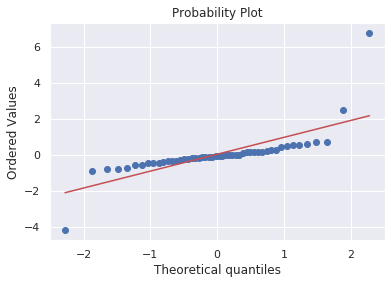In :
probplot(ts, dist='norm', plot=plt);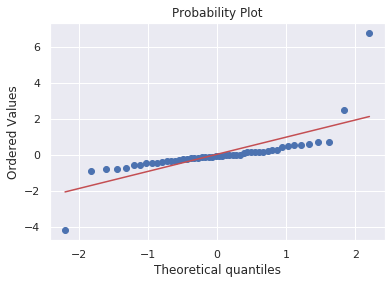### Another example¶

In :
df = sm.datasets.get_rdataset('iris').data
df.columns = [name.replace('.', '_').lower() for name in df.columns]  # Make name pythonic

df.describe()

Out:
sepal_length sepal_width petal_length petal_width
count 150.000000 150.000000 150.000000 150.000000
mean 5.843333 3.057333 3.758000 1.199333
std 0.828066 0.435866 1.765298 0.762238
min 4.300000 2.000000 1.000000 0.100000
25% 5.100000 2.800000 1.600000 0.300000
50% 5.800000 3.000000 4.350000 1.300000
75% 6.400000 3.300000 5.100000 1.800000
max 7.900000 4.400000 6.900000 2.500000
In :
df.isnull().any()  # Check if there is any missing value

Out:
sepal_length    False
sepal_width     False
petal_length    False
petal_width     False
species         False
dtype: bool
In :
import statsmodels.formula.api as smf

model =  smf.ols('sepal_length ~ petal_length + petal_width', data=df)
res = model.fit()
res.summary()

Out:
Dep. Variable: R-squared: sepal_length 0.766 OLS 0.763 Least Squares 241.0 Tue, 22 Sep 2020 4.00e-47 16:59:29 -75.023 150 156.0 147 165.1 2 nonrobust
coef std err t P>|t| [0.025 0.975] 4.1906 0.097 43.181 0.000 3.999 4.382 0.5418 0.069 7.820 0.000 0.405 0.679 -0.3196 0.160 -1.992 0.048 -0.637 -0.002
 Omnibus: Durbin-Watson: 0.383 1.826 0.826 0.54 0.06 0.763 2.732 25.3

Warnings:
 Standard Errors assume that the covariance matrix of the errors is correctly specified.

StatsModels accepts categorical variables:

In :
model = smf.ols('sepal_length ~ petal_length + petal_width + C(species)', data=df)
res = model.fit()
res.summary()

Out:
Dep. Variable: R-squared: sepal_length 0.837 OLS 0.832 Least Squares 185.8 Tue, 22 Sep 2020 5.33e-56 16:59:29 -48.116 150 106.2 145 121.3 4 nonrobust
coef std err t P>|t| [0.025 0.975] 3.6830 0.107 34.291 0.000 3.471 3.895 -1.5984 0.206 -7.770 0.000 -2.005 -1.192 -2.1126 0.304 -6.949 0.000 -2.714 -1.512 0.9059 0.074 12.191 0.000 0.759 1.053 -0.0060 0.156 -0.038 0.969 -0.315 0.303
 Omnibus: Durbin-Watson: 0.587 1.802 0.746 0.679 0.142 0.712 2.832 61.5

Warnings:
 Standard Errors assume that the covariance matrix of the errors is correctly specified.

To regress a variable y on all others, there is no notation equivalent to y ~ . in R. Instead, you can use the following workaround.

In :
def all_predictors(df, outcome):
return outcome + " ~ " + " + ".join(df.columns.difference([outcome]))

print(all_predictors(df, "sepal_length"))

sepal_length ~ petal_length + petal_width + sepal_width + species


## ANOVA ¶

The last linear model can be formalized as: $$Y = \mu \mathbb 1 + X\beta + A \alpha + \epsilon, \qquad s.t.~ \alpha_1 = 0,$$ where

• $Y \in \mathbb R^n$ is the vector of observations (sepal_length);
• $\mu \in \mathbb R$ is the intercept;
• $X \in \mathbb R^{n \times 2}$ is the design matrix;
• $\beta \in \mathbb R^2$ is the vector of coefficients (petal_length and petal_width);
• $A \in \mathbb R^{n \times 3}$ is the indicator matrix corresponding to the categorical variable (species);
• $\alpha \in \mathbb R^3$ is the vector of effects of the categorical values setosa, versicolor and virginica;
• $\epsilon \sim \mathcal N(0, \sigma^2 I_n)$ is a random noise.

An analysis of variance (in its generalized version) tests the significance of continuous and categorical variables (based on a Fisher test), that is the null hypotheses (see the ANOVA table below):

• $\alpha_1 = \alpha_2 = \alpha_3 = 0$ (significance of species);
• $\beta_1 = 0$ (significance of petal_length);
• $\beta_2 = 0$ (significance of petal_width).
In :
sm.stats.anova_lm(res)  # ANOVA table

Out:
df sum_sq mean_sq F PR(>F)
C(species) 2.0 63.212133 31.606067 274.728446 4.785160e-50
petal_length 1.0 22.274541 22.274541 193.616313 1.718161e-28
petal_width 1.0 0.000169 0.000169 0.001472 9.694469e-01
Residual 145.0 16.681489 0.115045 NaN NaN

We read the p-values in the last column. For the species, we observe a p-value that is small enough to reject the null hypothesis that the species does not impact the model. In the same manner, we can conclude that petal_length is an important factor (its coefficient is non-zero). However, the p-value for petal_width is large enough to conclude that, given the covariates species and petal_length, the variable petal_width does not provide an additional information, that explains the outcome sepal_length (it has no effect on the response variable).

Assume now that you know $\beta$ and consider the model: $$\tilde Y = Y - X\beta = \mu + A \alpha + \epsilon, \qquad s.t.~ \alpha_1 = 0.$$ The impact of the categorical variable species can be illustrated on the following graph, showing that the means are different according to the modalities of species (setosa, versicolor, virginica).

In :
# Observations \tilde Y
sepal_beta = (0.9059, -0.006)
observations = df['sepal_length'] - sepal_beta*df['petal_length'] - sepal_beta*df['petal_width']

plt.plot(observations[df['species']=='setosa'], label="setosa")
plt.plot(observations[df['species']=='versicolor'], label="versicolor")
plt.plot(observations[df['species']=='virginica'], label="virginica")
plt.legend(loc='best');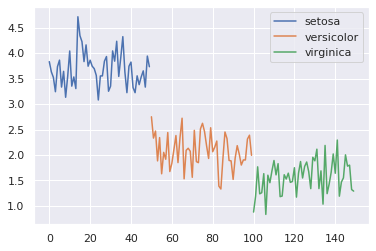Assume now that you would like to test the Gaussian assumption of the model, that is for each modality indexed by $j \in \{1, 2, 3\}$, observations are distributed according to $\mathcal N(\mu + \alpha_j, \sigma^2)$. You should test if the groups:

1. are normally distributed;
2. with equal variance.
In :
from scipy.stats import levene, bartlett, normaltest

for modality in ['setosa', 'versicolor', 'virginica']:
print(normaltest(observations[df['species']==modality]))

NormaltestResult(statistic=4.053279083071296, pvalue=0.13177761110864925)
NormaltestResult(statistic=0.7548077096205367, pvalue=0.6856391193186202)
NormaltestResult(statistic=0.11510513766630709, pvalue=0.944072260304799)


Samples can be considered normaly distributed. What about the variances?

In :
print(levene(observations[df['species']=='setosa'], observations[df['species']=='versicolor'],
observations[df['species']=='virginica']))
print(bartlett(observations[df['species']=='setosa'], observations[df['species']=='versicolor'],
observations[df['species']=='virginica']))

LeveneResult(statistic=0.14161247465879312, pvalue=0.8680758119020389)
BartlettResult(statistic=0.22506978073272027, pvalue=0.8935661697135951)


Variances can be considered the same.

# Exercises ¶

## Exercise 1 ¶

Draw a sample from a chi-squared distribution with 5 degrees of freedom. Plot the histogram and the probability distribution function on the same figure.

In [ ]:
# Answer


## Exercise 2 ¶

Generate a sample from a standard normal distribution and another from a gamma distribution with shape $a=\frac 14$ and scale $\theta=2$. Test for the null hypothesis that both samples have equal variances.

In [ ]:
# Answer


## Exercise 3 ¶

Illustrate the central limit theorem with a binomial distribution with parameters $n=10$ and $p=0.7$. Perform a test against a standard normal distribution to support the result.

In [ ]:
# Answer


## Exercise 4 ¶

This exercise proposes to analyse a dataset.

• Load the R dataset airquality with StatsModels utilities.
• Make columns names pythonic (remove "." from solar.m).
• Drop rows with missing data.
• Display a summary of the dataset.
• Perform a linear regression to explain the ozone variable with solar_r , wind, temp and month (categorical variable).
• Display a summary of the linear regression.
• Are the studentized residuals normaly distributed?
• Perform an ANOVA.
• Predict the air quality for today (suppose that solar_r = 207.0, convert wind to miles per hour and temp to degrees Fahrenheit by hitting Show nonmetric). Use res.predict(dict(solar_r=207, …)).
In [ ]:
# Answer


Implement a Metropolis Hastings algorithm. Use it to sample a beta distribution with parameters $\alpha=2$ and $\beta=5$. Compare with what is obtained with Scipy routines.
# Answer